# NCERT Solutions for Class 9 Maths Chapter 1 Number Systems| PDF Download

On this page you will get NCERT Solutions for Class 9 Maths Chapter Number Systems that will comfort you as you will get to know various concepts needed for solving various questions. These NCERT Solutions are updated as per the latest pattern of 2020-21 syllabus. Chapter 1 Class 10 Maths NCERT Solutions PDF Download can be really helpful if anyone want to understand the detailed solutions and minimise the errors wherever possible.

For better understanding and application of concepts, one should first need to focus on the Number Systems NCERT Solutions as it will tell you about the difficulty of questions. You can be able to solve more questions related to chapter after practising more questions and thus fetching more marks.Page No: 5

Exercise 1.1

1. Is zero a rational number? Can you write it in the form p/q, where p and q are integers and q ≠ 0?

Yes. Zero is a rational number as it can be represented as 0/1 or 0/2.

2. Find six rational numbers between 3 and 4.

There are infinite rational numbers in between 3 and 4.
3 and 4 can be represented as 24/8 and 32/8 respectively.
Therefore, six rational numbers between 3 and 4 are
25/8, 26/8, 27/8, 28/8, 29/8, 30/8.

3. Find five rational numbers between 3/5 and 4/5.

There are infinite rational numbers in between 3/5 and 4/5
3/5 = 3×6/5×6 = 18/30
4/5 = 4×6/5×6 = 24/30
Therefore, five rational numbers between 3/5 and 4/5 are
19/30, 20/30, 21/30, 22/30, 23/30.

4. State whether the following statements are true or false. Give reasons for your answers.

(i) Every natural number is a whole number.
► True, since the collection of whole numbers contains all natural numbers.

(ii) Every integer is a whole number.
► False, as integers may be negative but whole numbers are always positive.

(iii) Every rational number is a whole number.
► False, as rational numbers may be fractional but whole numbers may not be.

Page No: 8

Exercise 1.2

1. State whether the following statements are true or false. Justify your answers.

(i) Every irrational number is a real number.
► True, since the collection of real numbers is made up of rational and irrational numbers.

(ii) Every point on the number line is of the form√m, where m is a natural number.
► False, since positive number cannot be expressed as square roots.

(iii) Every real number is an irrational number.
► False, as real numbers include both rational and irrational numbers. Therefore, every real number cannot be an irrational number.

2. Are the square roots of all positive integers irrational? If not, give an example of the square root of a number that is a rational number.

No, the square roots of all positive integers are not irrational. For example √4 = 2.

3. Show how √5 can be represented on the number line.

Step 1: Let AB be a line of length 2 unit on number line.
Step 2: At B, draw a perpendicular line BC of length 1 unit. Join CA.
Step 3: Now, ABC is a right angled triangle. Applying Pythagoras theorem,
AB2 + BC2 = CA2
⇒ 22 + 12 = CA2
⇒ CA2 = 5
⇒ CA = √5
Thus, CA is a line of length √5 unit.
Step 4: Taking CA as a radius and A as a centre draw an arc touching
the number line. The point at which number line get intersected by
arc is at √5 distance from 0 because it is a radius of the circle
whose centre was A.
Thus, √5 is represented on the number line as shown in the figure.Page No: 14

Exercise 1.3

1. Write the following in decimal form and say what kind of decimal expansion each has:
(i) 36/100
= 0.36 (Terminating)

(ii) 1/11
0.09090909... = 0.9 (Non terminating repeating)

= 33/8 = 4.125 (Terminating)

(iv) 3/13
= 0.230769230769... = 0.230769 (Non terminating repeating)

(v) 2/11
= 0.181818181818... = 0.18 (Non terminating repeating)

(vi) 329/400
= 0.8225 (Terminating)

2. You know that 1/7 = 0.142857.Can you predict what the decimal expansion of 2/7, 3/7, 4/7, 5/7, 6/7 are without actually doing the long division? If so, how?
[Hint: Study the remainders while finding the value of 1/7 carefully.]

Yes. We can be done this by: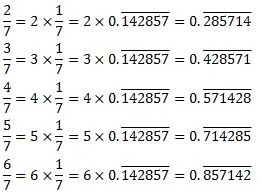3. Express the following in the form p/q where p and q are integers and q ≠ 0.
(i) 0.6
(ii) 0.47
(iii) 0.001

(i) 0.6 = 0.666...
Let x = 0.666...
10x = 6.666...
10x = 6 + x
9x = 6
x = 2/3

(ii) 0.47 = 0.4777...
= 4/10 + 0.777/10
Let x = 0.777…
10x = 7.777…
10x = 7 + x
x = 7/9
4/10 + 0.777.../10 = 4/10 + 7/90
= 36/90 +7/90 = 43/90

(iii) 0.001 = 0.001001...
Let x = 0.001001...
1000x = 1.001001…
1000x = 1 + x
999x = 1
x = 1/999

4. Express 0.99999…in the form  p/q. Are you surprised by your answer? With your teacher and classmates discuss why the answer makes sense.

Let x = 0.9999…
10x = 9.9999…
10x = 9 + x
9x = 9
x = 1
The difference between 1 and 0.999999 is 0.000001 which is negligible. Thus, 0.999 is too much near 1, Therefore, the 1 as answer can be justified.

5. What can the maximum number of digits be in the repeating block of digits in the decimal expansion of 1/17? Perform the division to check your answer.

1/17 = 0.0588235294117647
There are 16 digits in the repeating block of the decimal expansion of 1/17.
Division Check: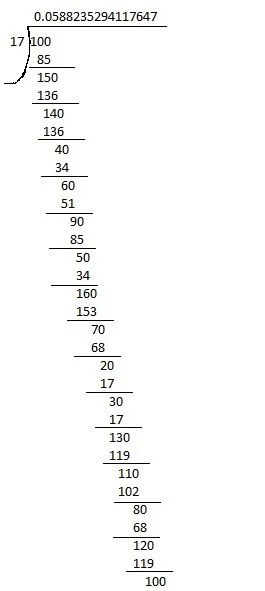= 0.0588235294117647

6. Look at several examples of rational numbers in the form p/(≠ 0) where p and q are integers with no common factors other than 1 and having terminating decimal representations (expansions). Can you guess what property q must satisfy?

We observe that when q is 2, 4, 5, 8, 10... then the decimal expansion is terminating. For example:
1/2 = 0.5, denominator q = 21
7/8 = 0.875, denominator q = 23
4/5 = 0.8, denominator q = 51

We can observed that terminating decimal may be obtained in the situation where prime factorisation of the denominator of the given fractions has the power of 2 only or 5 only or both.

7. Write three numbers whose decimal expansions are non-terminating non-recurring.

Three numbers whose decimal expansions are non-terminating non-recurring are:
0.303003000300003...
0.505005000500005...
0.7207200720007200007200000…

8. Find three different irrational numbers between the rational numbers 5/7 and 9/11.

5/7 = 0.714285
9/11 = 0.81
Three different irrational numbers are:
0.73073007300073000073…
0.75075007300075000075…
0.76076007600076000076…

9. Classify the following numbers as rational or irrational:
(i) √23
(ii) √225
(iii) 0.3796
(iv) 7.478478
(v) 1.101001000100001…

(i) √23 = 4.79583152331...
Since the number is non-terminating non-recurring therefore, it is an irrational number.

(ii) √225 = 15 = 15/1
Since the number is rational number as it can represented in p/q form.

(iii) 0.3796
Since the number is terminating therefore, it is an rational number.

(iv) 7.478478 = 7.478
Since the this number is non-terminating recurring, therefore, it is a rational number.

(v) 1.101001000100001…
Since the number is non-terminating non-repeating, therefore, it is an irrational number.

Page No: 18

Exercises 1.4

1. Visualise 3.765 on the number line using successive magnification.2. Visualise 4.26 on the number line, up to 4 decimal places.

4.26 = 4.2626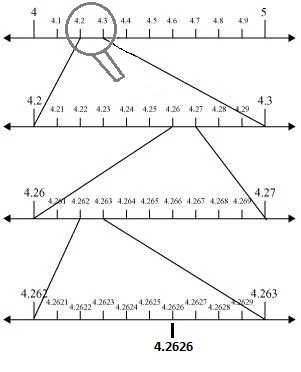Page No: 24

Exercise 1.5

1. Classify the following numbers as rational or irrational:
(i) 2 - √5
(ii) (3 + √23) - √23
(iii) 2√7/7√7
(iv) 1/2
(v) 2π

(i) 2 - √5 = 2 - 2.2360679… = - 0.2360679…
Since the number is is non-terminating non-recurring therefore, it is an irrational number.

(ii) (3 + √23) - √23 = 3 + √23 - √23 = 3 = 3/1
Since the number is rational number as it can represented in p/form.

(iii) 2√7/7√7 = 2/7
Since the number is rational number as it can represented in p/form.

(iv) 1/2 = √2/2 = 0.7071067811...
Since the number is is non-terminating non-recurring therefore, it is an irrational number.

(v) 2π = 2 × 3.1415… = 6.2830…
Since the number is is non-terminating non-recurring therefore, it is an irrational number.

2. Simplify each of the following expressions:

(i) (3 + √3) (2 + √2)
(ii) (3 + √3) (3 - √3)
(iii) (√5 + √2)2
(iv) (√5 - √2) (√5 + √2)

(i) (3 + √3) (2 + √2)
⇒ 3 × 2 + 2 + √3 + 3√2+ √3 ×√2
⇒ 6 + 2√3 +3√2 + √6

(ii) (3 + √3) (3 - √3) [∵ (a + b) (a - b) = a2 - b2]
⇒ 32 - (√3)2
⇒ 9 - 3
⇒ 6

(iii) (√5 + √2)[∵ (a + b)2 = a2 + b2 + 2ab]
⇒ (√5)2 + (√2)2 + 2 ×√5 × √2
⇒ 5 + 2 + 2 × √5× 2
⇒ 7 +2√10

(iv) (√5 - √2) (√5 + √2) [∵ (a + b) (a - b) = a2 - b2]
⇒ (√5)2 - (√2)2
⇒ 5 - 2
⇒ 3

3. Recall, π is defined as the ratio of the circumference (say c) of a circle to its diameter (say d). That is, π = c/d. This seems to contradict the fact that π is irrational. How will you resolve this contradiction?

There is no contradiction. When we measure a value with a scale, we only obtain an approximate value. We never obtain an exact value. Therefore, we may not realise that either c or d is irrational. The value of π is almost equal to 22/7 or 3.142857...

4. Represent √9.3 on the number line.

Step 1: Draw a line segment of unit 9.3. Extend it to C so that BC is of 1 unit.
Step 2: Now, AC = 10.3 units. Find the centre of AC and name it as O.
Step 3: Draw a semi circle with radius OC and centre O.
Step 4: Draw a perpendicular line BD to AC at point B which intersect the semicircle at D. Also, Join OD.
Step 5: Now, OBD is a right angled triangle.
Here, OD = 10.3/2 (radius of semi circle), OC = 10.3/2, BC = 1
OB = OC – BC = (10.3/2) – 1 = 8.3/2
Using Pythagoras theorem,
OD2 = BD2 + OB2
⇒ (10.3/2)2 = BD2 + (8.3/2)2
⇒ BD2 = (10.3/2)2 - (8.3/2)2
⇒ BD2 = (10.3/2 – 8.3/2) (10.3/2 + 8.3/2)
⇒ BD2 = 9.3
⇒ BD2 =  √9.3
Thus, the length of BD is √9.3.
Step 6: Taking BD as radius and B as centre draw an arc which touches the line segment. The point where it touches the line segment is at a distance of √9.3 from O as shown in the figure.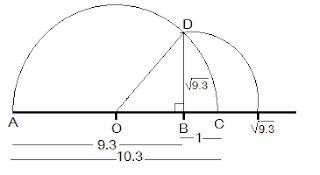5. Rationalise the denominators of the following:
(i) 1/7
(ii) 1/7-√6
(iii) 1/5+√2
(iv) 1/7-2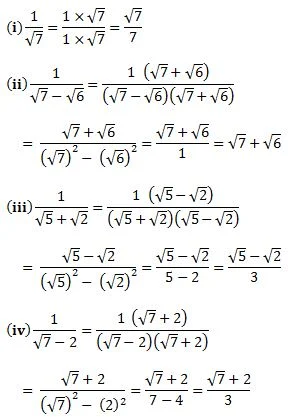Page No: 26

Exercise 1.6

1. Find:
(i) 641/2
(ii) 321/5
(iii) 1251/32. Find:
(i) 93/2
(ii) 322/5
(iii) 163/4
(iv) 125-1/3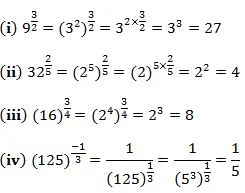3. Simplify:
(i) 22/3.21/5
(ii) (1/33)7
(iii) 111/2/111/4
(iv) 71/2.81/2Go Back to NCERT Solutions for Class 9 Maths

## NCERT Solutions for Class 9 Maths Chapter 1 Number Systems

Number Systems is the first chapter of Class 9 Maths textbook in which we study about natural numbers, whole numbers and integers. This chapter details about rational and irrational numbers. A number ‘r’ is called a rational number, if it can be written in the form p/q, where p and q are integers and q ≠ 0.

• Irrational Numbers: A number ‘s’ is called irrational, if it cannot be written in the form p/q, where p and q are integers and q ≠ 0. The collection of all rational numbers and irrational numbers together make up what we call the collection of real numbers. Every real number is represented by a unique point on the number line.

• Real Numbers and their Decimal Expansions: We will look at the decimal expansions of real numbers and see if we can use the expansions to distinguish between rationals and irrationals and also explain how to visualise the representation of real numbers on the number line using their decimal expansions. The decimal expansion of a rational number is either terminating or nonterminating recurring. The decimal expansion of an irrational number is non-terminating non-recurring

• Representing Real Numbers on the Number Line: The process of visualisation of representation of numbers on the number line, through a magnifying glass is known as the process of successive magnification. Every real number is represented by a unique point on the number line. Further, every point on the number line represents one and only one real number.

• Operations on Real Numbers: We will be dealing with multiple operations like addition, subtraction, multiplication and division of irrational numbers.

• Laws of Exponents for Real Numbers: We will use laws of exponents to solve the questions given in the exercise.

There are total 6 exercises in the chapter which will be help you in revising the chapter properly. We have provided Class 9 Chapter 1 Maths NCERT Solutions step by step on Studyrankers. You only need to click on the exercises given below and start clearing your doubts.

Studyrankers experts have prepared Chapter 1 Class 9 Maths NCERT Solutions in well manner so a student can easily clear their misunderstandings any time.

### NCERT Solutions for Class 9 Maths Chapters:

FAQ on Chapter 1 Number Systems

#### Why we should solve NCERT Solutions for Chapter 1 Number Systems Class 9?

Chapter 1 Number Systems Class 9 Maths is very important for every student as it will help you in understanding what is given in the chapter and understand the concepts. It is very essential to solve every question before moving further to any other supplementary books.

–1, 0 and 1

#### Find the sum of  (3√3 +7√2) and (√3 - 5√2).

We have  (3√3 +7√2) + (√3 - 5√2)
= √3 3+7√2 + √3 - 5√2
= (3√3+√3) + 7√2 - 5√2)
=√3(3+1) + √2(7-2)
=√3(4) + √2(5) = (4√3 + 2√2)

#### How can I download PDF of Chapter 1 Number Systems Class 9 NCERT Solutions?

You can easily download PDF of Class 9 NCERT Solutions Chapter 1 here through which you can easily get what you're looking for.